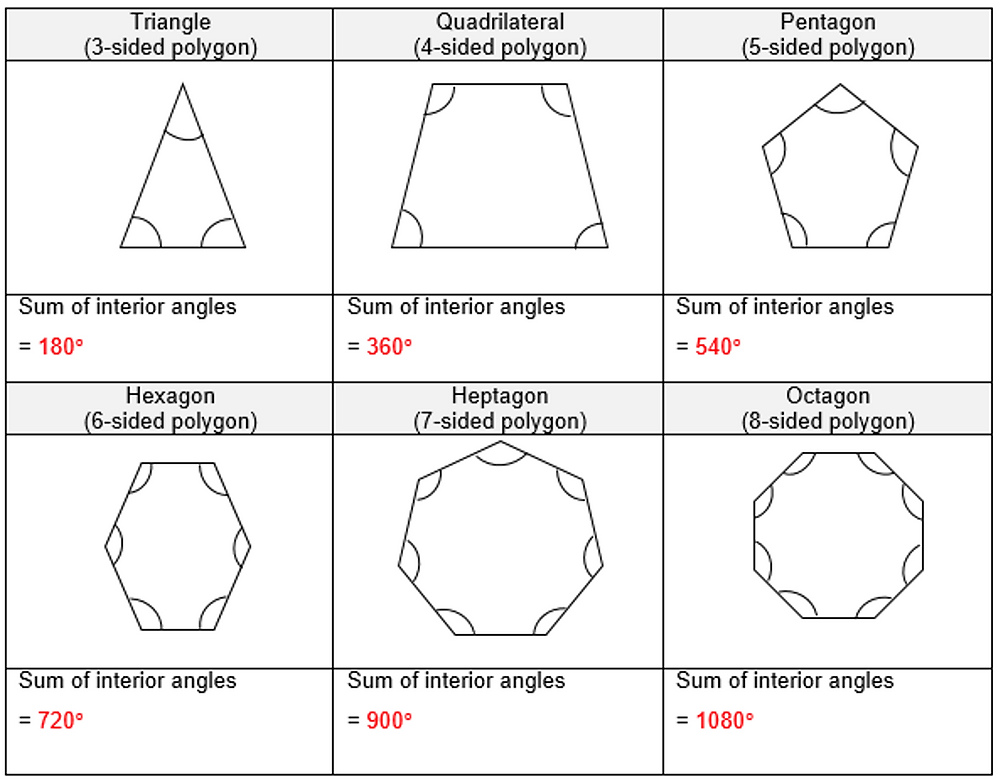Search

# Providing opportunities to discover Maths (Inductive & Deductive Reasoning) - Joyous Maths Pt. 4

Inductive Reasoning

Optimum scaffolding allows students to arrive at mathematical rules and formulae themselves. Not only does this help them remember the rules better, it also makes Maths fun and rewarding! This point is aptly illustrated through Extract G (part 1), taken from a Joyous Learning Primary Six worksheet on Geometry.

Extract G (part 1)

Polygons

The Oxford English Dictionary defines “polygon” as “a plane figure with at least three straight sides and angles, and typically five or more”. The word itself is derived from Greek where “poly” means “many” and “gon” means “angle” or “corner”.

In today’s lesson, you have encountered 3-sided (triangles) and 4-sided (squares, rectangles, trapeziums, rhombus and parallelograms) polygons. The general name for 4-sided polygons is quadrilateral. In fact, you have also worked with 5- and 6-sided polygons in the form of composite figures made up of 3- and 4-sided polygons. Let’s discover more about polygons!

Measure and find the sum of all the interior angles of each polygon.Observe that each successive polygon’s sum of interior angles increases by 180°. Try to imagine: the delight felt by the student at the point of discovering this for himself! This sense of satisfaction of that Eureka moment beats any toy promised as a reward to do Maths!Besides embedding optimum scaffolding in the teaching materials, a skillful teacher is indispensable to guiding students in this discovery process.

Of course, this is not to say that we have to start throwing the formula books out of the window, which brings us to the next point on deductive reasoning in Maths.

Deductive Reasoning

The sum of all interior angles in a triangle is usually given to students as a rule. This is followed by applying the standard rule to find specific unknown angles in polygons, usually quadrilaterals. These are good processes to follow but more can be done to stretch students in terms of applying mathematical rules, or deductive reasoning. Have a look at Extract G (part 2), a continuation of the previous extract.

Extract G (part 2)

There is another way to understand the above pattern. Refer to the quadrilateral below. A vertex is a point where two sides of a polygon meet to form an interior angle. The number of vertices a polygon has corresponds to the number of sides it has. The dotted line cuts through two vertices of the quadrilateral to produce two triangles. Now, remember that the sum of interior angles of a triangle is 180°, so the sum of the interior angles of two triangles is 360°, which is precisely the sum of the interior angles of a quadrilateral!Go on; try this method out on other polygons. One more thing … keep in mind that your “dotted line” must cut through two vertices of the polygon and these two vertices cannot be next to each other. Have fun!

Essentially, what students are doing here is applying a rule (sum of interior angles of a triangle) that they have been taught, to understand another rule (sum of interior angles of a polygon). In other words, they are learning how the rule of sum of interior angles of a polygon is derived from or is built upon the rule of sum of interior angles of a triangle.

Providing students with such opportunities enhances their understanding of what is taught and reduces mindless memorisation.